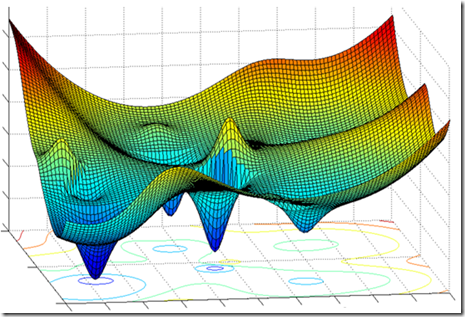Want to share your content on R-bloggers? click here if you have a blog, or here if you don't.

Here below you can find the multivariable, (2 variables version) of the gradient descent algorithm. You could easily add more variables. For sake of simplicity and for making it more intuitive I decided to post the 2 variables case. In fact, it would be quite challenging to plot functions with more than 2 arguments.

Say you have the function f(x,y) = x**2 + y**2 –2*x*y plotted below (check the bottom of the page for the code to plot the function in R):Well in this case, we need to calculate two thetas in order to find the point (theta,theta1) such that f(theta,theta1) = minimum.

Here is the simple algorithm in Python to do this:

This function though is really well behaved, in fact, it has a minimum each time x = y. Furthermore, it has not got many different local minimum which could have been a problem. For instance, the function here below would have been harder to deal with.Finally, note that the function I used in my example is again, convex.